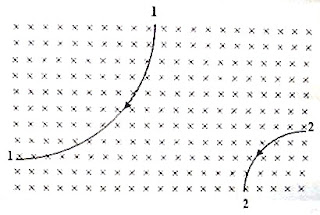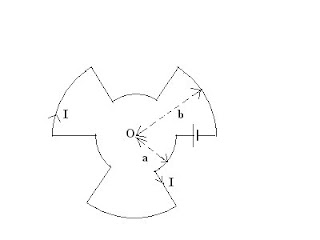## Pages

`“Life is like riding a bicycle.  To keep your balance you must keep moving.”–Albert Einstein`

## Sunday, May 2, 2010

### AP Physics B & C – Magnetic Fields – Multiple Choice Practice Questions

Questions involving magnetic fields were discussed on many occasions on this blog. You can access them by trying a search for ‘magnetic field’ using the search box provided on this page or by clicking on the label ‘magnetic field’ below this post.
The following multiple choice practice questions involving magnetic fields will be worth noting even if you find very limited time for your final preparation for the fast approaching AP Physics B as well as AP Physics C examination:(1) The adjoining figure shows the paths of two particles (1 and 2) carrying charges of the same magnitude, when projected, with the same speed, at right angles to a uniform magnetic field. The magnetic field is directed normally into the plane of the figure. Pick out the correct statement from the following:

(a) Particle 1 is negatively charged, particle 2 is positively charged and particle 2 is heavier than particle 1.

(b) Particle 1 is negatively charged, particle 2 is positively charged and particle 1 is heavier than particle 2.

(c) Particle 1 and particle 2 are positively charged and particle 2 is heavier than particle 1.

(d) Particle 1 and particle 2 are negatively charged and particle 2 is heavier than particle 1.

(e) Particle 1 is positively charged, particle 2 is negatively charged and particle 1 is heavier than particle 2.

Since the amounts of charges are equal, the magnetic forces in the uniform field are of equal magnitude. So the radius of curvature of the heavier particle is greater, which is the case with particle 1.

[We have mv2/r = qvB, on equating the centripetal force to the magnetic force so that the radius of the path is given by

r = mv/qB

Since v, q and B are the same, r must be greater for the heavier particle].

On applying Fleming’s left hand rule (motor rule) to particle 2 we obtain the sense of bending as expected for a positively charged particle.

[Hold your fore-finger along the direction of the magnetic field and the thumb along the direction of bending (direction of magnetic force). If the direction of motion of the particle is representable by the middle finger, the particle is positively charged. Otherwise, it is negatively charged].

Particle 1 is found to be negatively charged on applying Fleming’s left hand rule. The correct option is (b).

(2) A proton is moving in a static uniform magnetic field. There are no other fields. Which one of the following statements is false?

(a) The kinetic energy of the proton remains unchanged

(b) The magnetic field can exert a force on the proton only if it is in motion.

(c) The magnetic field changes the velocity of the proton.

(d) The magnetic field reduces the speed of the proton

(e) The magnetic field does not change the kinetic energy of the particle.

Since the magnetic force on the proton is always at right angles to its displacement, no work is done by the magnetic field. It can just deflect the proton, thereby changing the direction of its velocity, without altering its magnitude (speed).

(3) Two long straight conductors C1 and C2 carrying the same current I flowing in the positive z-direction are arranged parallel to the z-axis of a right handed Cartesian coordinate system. The conductors are at the same distance s from the z-axis, as shown in the figure. What is the magnitude of the resultant magnetic flux density at the origin?

(a) μ0I/πs

(b) μ0I/2πs

(c) √2 (μ0I/πs)

(d) (1/√2)(μ0I/πs)

(e) zero

Conductor C1 and C2 carrying the current I produce magnetic fields of the same magnitude (B = μ0I/2πs) at the origin O. These fields are at right angles to each other and hence the resultant field at the origin is [(μ0I/2πs)2 + (μ0I/2πs)2 ]1/2 = √2 0I/2πs) = (1/√2)(μ0I/πs), as given in option (c).

[Note that the field at the origin due to C1 is along the positive y-direction where as the field at the origin due to C2 is along the positive x-direction. The resultant field is inclined at 45º with the positive x-direction and the positive y-direction].

(4) A time varying magnetic field is applied in a region of space where a stationary electron and a moving electron are present. Ignore the interaction between the electrons (as they are sufficiently farther apart). Then

(a) the stationary electron and the moving electron are unaffected.

(b) the stationary electron and the moving electron experience forces.

(c) the stationary electron is unaffected where as the moving electron experiences forces.

(d) the moving electron is unaffected where as the stationary electron experiences forces.

(e) the moving electron comes to rest where as the stationary electron starts moving.

The correct option is (b) since a changing magnetic field will produce an induced emf and the stationary electron and the moving electron will experience forces.

The following question is meant for AP Physics C aspirants; but AP Physics B aspirants too can try to solve it:(5) A conductor is bent in the form shown in the adjoining figure so that there are six straight radial portions of equal length and six concentric arcs of circles of radii ‘a’ and ‘b’, subtending the same angle at the common centre O. A battery connected in the circuit drives a steady current ‘I’ through the circuit. What is the magnitude of the magnetic flux density at the centre O?

(a) μ0I(a+b)/4ab

(a) μ0I(a+b)/3ab

(c) μ0I(a+b)/2ab

(d) μ0I(a+b)/ab

(e) Zero

The straight radial portions will not produce any magnetic field at the centre O. The field at the centre is produced by three identical arcs of a circle of radius ‘a’ and three identical arcs of a circle of radius ‘b’. The three smaller arcs will make a semicircle of radius ‘a’ and the three larger arcs will make a semicircle of radius ‘b’. The magnetic fields produced by them are in the same direction (acting normally into the plane of the figure) and hence they add up to produce a resultant field at the centre O.

Therefore, the magnetic field B at the centre O is given by

B = ½ × μ0I/2a + ½ × μ0I/2b

[The factor ½ appears because the fields are due to semicircular currents].

Thus B = (μ0I/4)(1/a + 1/b) = μ0I(a+b)/4ab

[Suppose we have a circuit of the form shown below: